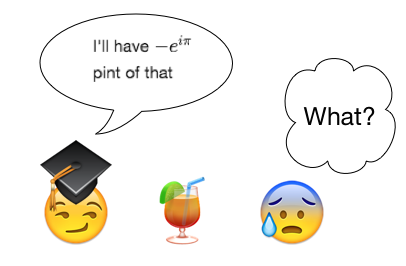# A Mathematician Went To A Bar

Algebra Level 1A mathematician went to a bar and ordered, "I'll have ${ -e }^{ i\pi }$ pint of beer."

How much (in pints) did he order?

×